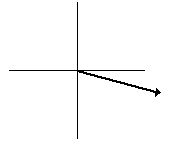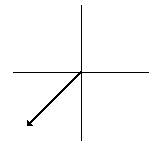# Vector Direction

This web page is designed to provide some additional practice with the use of scaled vector diagrams for the representation of the magnitude and direction of a vector. Your time will be best spent if you read each practice problem carefully, attempt to solve the problem, and then check your answer. You are cautioned to avoid making a quick reference to the solution prior to making your own attempt at the solution. Such a habit is likely to fail at nurturing the ability to draw a scaled vector diagram. If the solution to these practice problems are still not meaningful, you are encouraged to obtain some on-line help in The Physics Classroom Tutorial - visit the page on vector direction.

Determine the magnitude and direction of the following vectors in Questions #1 - #6. Use the counter-clockwise (from East) convention discussed in class to determine the direction. Use the indicated scale and a scale conversion to determine the magnitude. Use the pull-down to view the answers.

1. Given the SCALE: 1 cm = 10 m/s, determine the magnitude and direction of this vector.2. Given the SCALE: 1 cm = 50 km/hr, determine the magnitude and direction of this vector.3. Given the SCALE: 1 cm = 10 m/s, determine the magnitude and direction of this vector.4. Given the SCALE: 1 cm = 50 km/hr, determine the magnitude and direction of this vector.5. Given the SCALE: 1 cm = 10 m/s, determine the magnitude and direction of this vector.6. Given the SCALE: 1 cm = 50 km/hr, determine the magnitude and direction of this vector.Use an accurately-drawn scaled vector diagram to represent the magnitude and direction of the following vectors in Questions #7 - #12. Use the indicated scale and the counter-clockwise convention discussed in class. Click on the hot link to check the answers.

NOTE: Since your answers were determined using a scaled vector diagram, small errors in the measurement of the direction and magnitude of any one of the vectors may lead to small differences between your answers and the correct ones which are shown here. This should not be a reason for concern.

7. Given the SCALE: 1 cm = 10 m, represent the vector 50 m, 30-degrees by a scaled vector diagram.

8. Given the SCALE: 1 cm = 10 m, represent the vector 60 m, 150-degrees by a scaled vector diagram.

9. Given the SCALE: 1 cm = 20 m, represent the vector 140 m/s, 200-degrees by a scaled vector diagram.